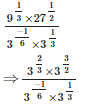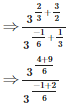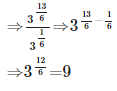# Test: Number Systems - 1

## 25 Questions MCQ Test Mathematics (Maths) Class 9 | Test: Number Systems - 1

Description
This mock test of Test: Number Systems - 1 for Class 9 helps you for every Class 9 entrance exam. This contains 25 Multiple Choice Questions for Class 9 Test: Number Systems - 1 (mcq) to study with solutions a complete question bank. The solved questions answers in this Test: Number Systems - 1 quiz give you a good mix of easy questions and tough questions. Class 9 students definitely take this Test: Number Systems - 1 exercise for a better result in the exam. You can find other Test: Number Systems - 1 extra questions, long questions & short questions for Class 9 on EduRev as well by searching above.
QUESTION: 1

### Find the remainder when 73 × 75 × 78 × 57 × 197 × 37 is divided by 34.

Solution:

Remainder,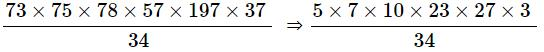[We have taken individual remainder, which means if 73 is divided by 34 individually, it will give remainder 5, 75 divided 34 gives remainder 7 and so on.]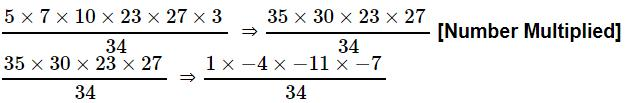[We have taken here negative as well as positive remainder at the same time. When 30 divided by 34 it will give either positive remainder 30 or negative remainder -4. We can use any one of negative or positive remainder at any time.]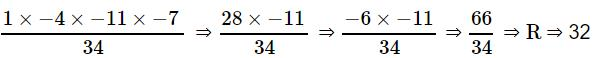Required remainder = 32.

QUESTION: 2

### If x = 2+√3, then x + 1/x =

Solution: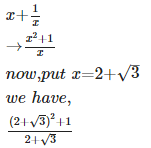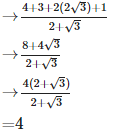QUESTION: 3

### The representation of octal number (532.2)8 in decimal is :

Solution:

Octal to Decimal conversion is obtained by multiplying 8 to the power of base index along with the value at that index position.

(532.2)8 = 5 * 82 + 3 * 81 + 2 * 80 + 2 * 8-1 = (346.25)10

QUESTION: 4

The value of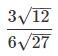is

Solution: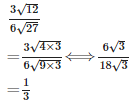QUESTION: 5

The value of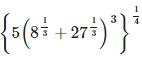is

Solution: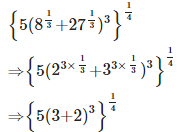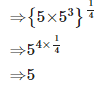QUESTION: 6

Which of the following is an rational number?

Solution:
QUESTION: 7

If 146! Is divisible by 5n, and then find the maximum value of n.

Solution: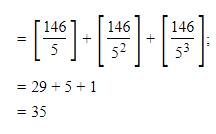Note:
We have taken integral value only, not the fractional.
For example 146/5 = 29.2 but we have taken 29 and so on.

QUESTION: 8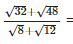Solution: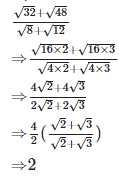QUESTION: 9

(5+√8)+(3−√2)(√2−6) is

Solution: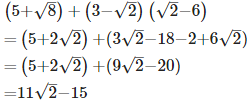And we know that the value of 11√2 is greater than 15 so it's value will be positive, And also sum or differences of rational and irrational is irrational

QUESTION: 10

√8+2√32−5√2 is equal to

Solution: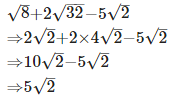QUESTION: 11

Every rational number is

Solution:

Every rational number is a real number. Real Number is a set of numbers formed by both Rational and Irrational numbers are combined.

QUESTION: 12

The simplest form of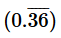is

Solution: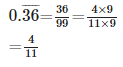QUESTION: 13

(125/216) -1/3 =

Solution: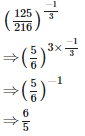QUESTION: 14

8√15 ÷ 2√3

Solution: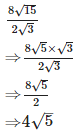QUESTION: 15

Ifn x = 3+2√2, then the value of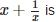Solution: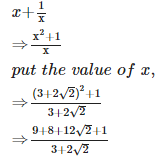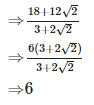QUESTION: 16

Decimal representation of a rational number cannot be

Solution:

A non-terminating, non-repeating decimal is a decimal number that continues endlessly, with no group of digits repeating endlessly.

Decimals of this type cannot be represented as fractions, and as a result, are irrational numbers.

QUESTION: 17

The simplest form of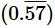is

Solution:
QUESTION: 18

If 3x + 64 = 26 + (√3)8, then the value of ‘x’ is

Solution: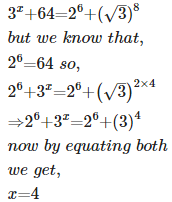QUESTION: 19

If x1/12 = 491/24, then the value of ‘x’ is

Solution: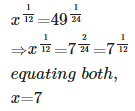QUESTION: 20

The value of (0.00032)-2/5 is

Solution: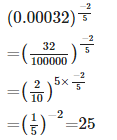QUESTION: 21

The decimal representation of an irrational number is

Solution:
QUESTION: 22

A number which can neither be expressed as a terminating decimal nor as a repeating decimal is called

Solution:
QUESTION: 23

The value of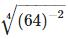is

Solution: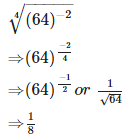QUESTION: 24

Which of the following is a rational number?

Solution:

0 is an integer and all integers are rational numbers.

QUESTION: 25

The value of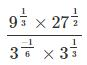is

Solution: